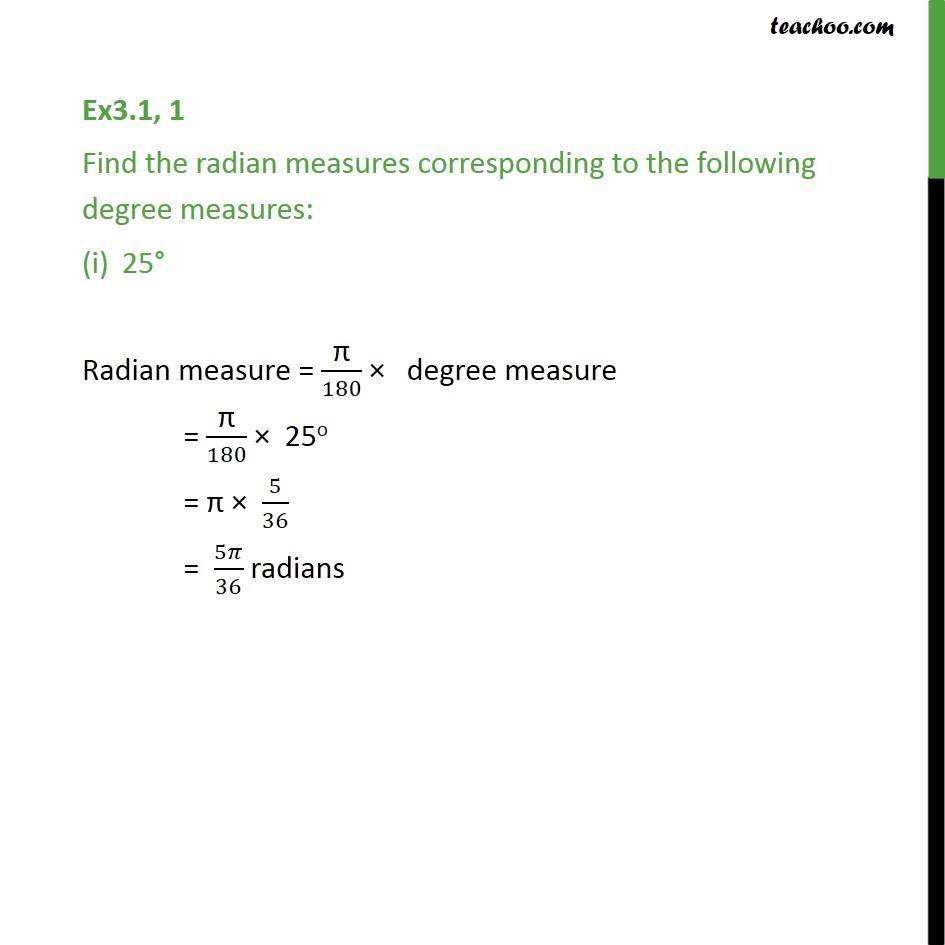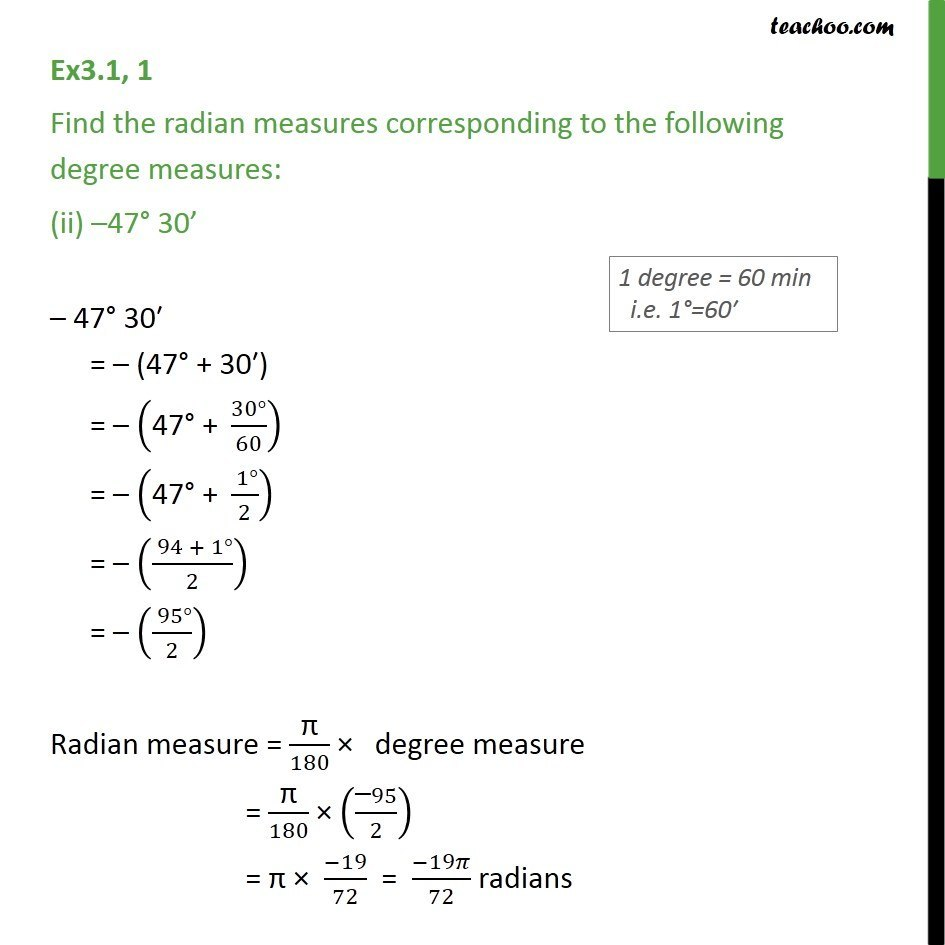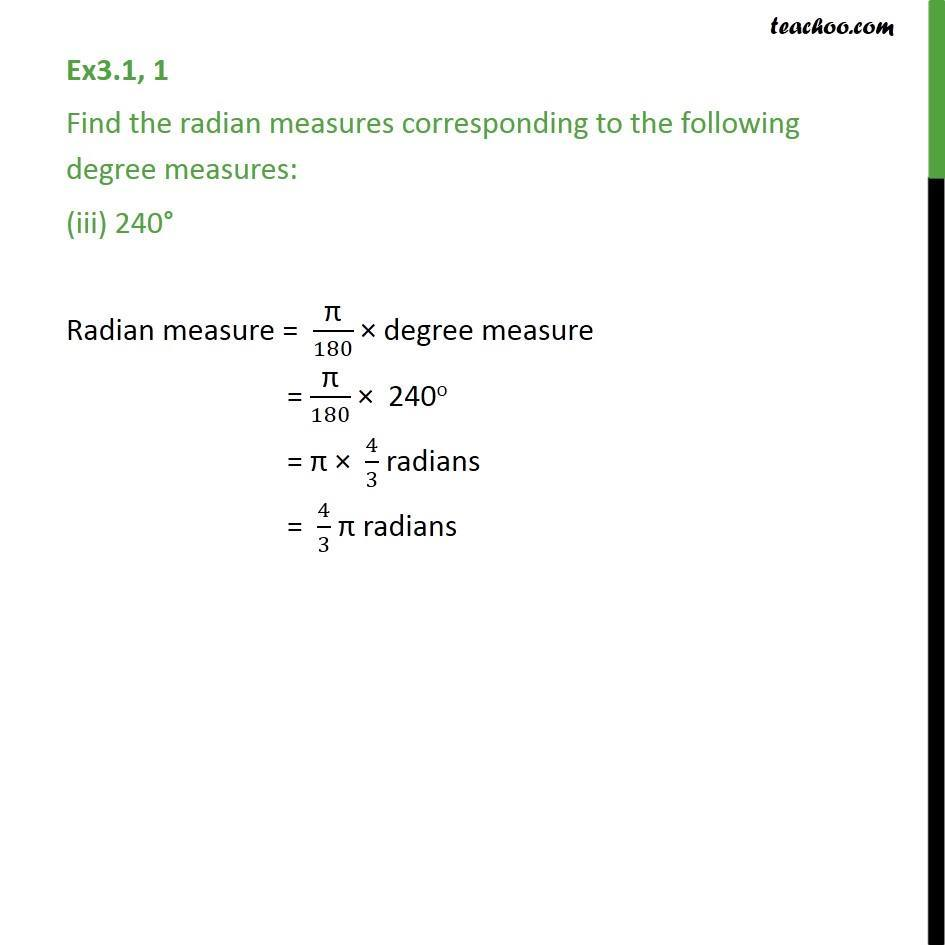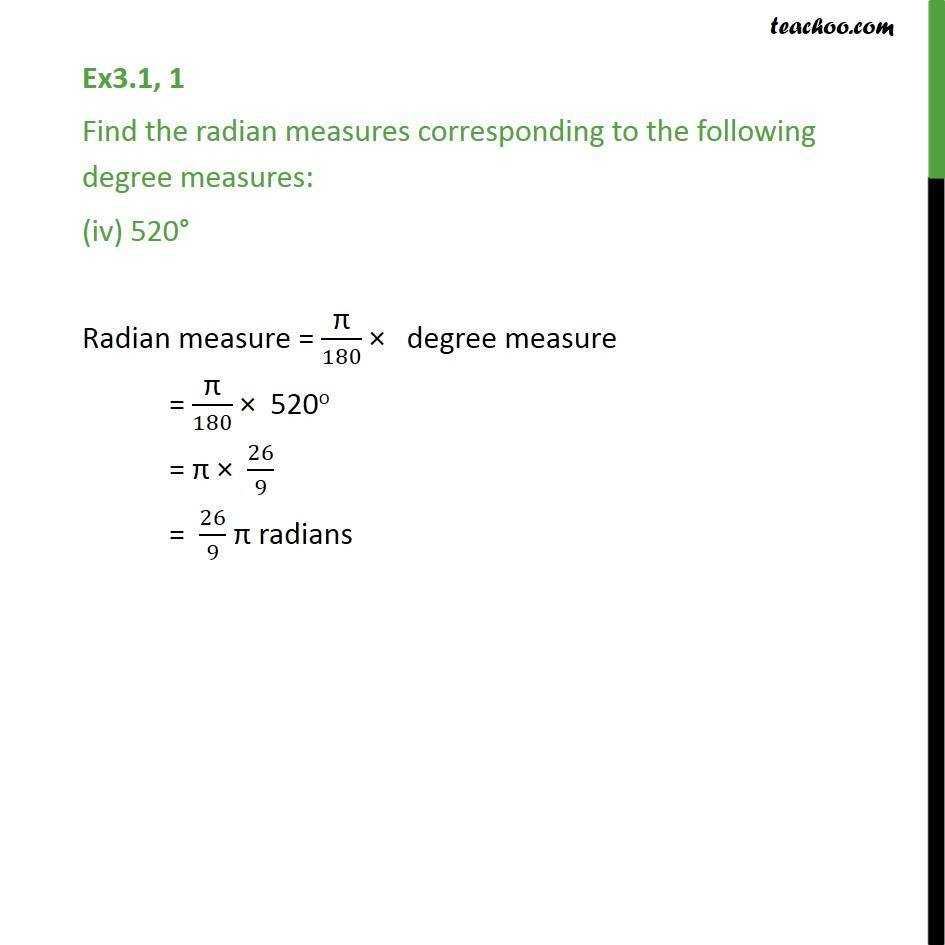1. Class 11
2. Important Question for exams Class 11
3. Chapter 3 Class 11 Trigonometric Functions

Transcript

Ex3.1, 1 Find the radian measures corresponding to the following degree measures: 25° Radian measure = "π" /180 × degree measure = "π" /180 × 25o = "π" × 5/36 = 5𝜋/36 radians Ex3.1, 1 Find the radian measures corresponding to the following degree measures: (ii) –47° 30’ – 47° 30′ = – (47° + 30′) = – ("47° + " (30°)/60) = – ("47° + " ( 1°)/2) = – (( 94 + 1°)/2) = – (( 95°)/2) Radian measure = "π" /180 × degree measure = "π" /180 × (("–" 95)/2) = "π" × (−19)/72 = (−19𝜋)/72 radians Ex3.1, 1 Find the radian measures corresponding to the following degree measures: (iii) 240° Radian measure = "π" /180 × degree measure = "π" /180 × 240o = "π" × 4/3 radians = 4/3 "π" radians Ex3.1, 1 Find the radian measures corresponding to the following degree measures: (iv) 520° Radian measure = "π" /180 × degree measure = "π" /180 × 520o = "π" × 26/9 = 26/9 "π" radians

Chapter 3 Class 11 Trigonometric Functions

Class 11
Important Question for exams Class 11

About the AuthorDavneet Singh
Davneet Singh is a graduate from Indian Institute of Technology, Kanpur. He has been teaching from the past 9 years. He provides courses for Maths and Science at Teachoo.